this notebook shows a model for a multinomial Simpson paradox.

In :

import matplotlib.pyplot as plt
import random

import pandas as pd
import numpy as np

import pyAgrum as gum
import pyAgrum.lib.notebook as gnb

import pyAgrum.causal as csl
import pyAgrum.causal.notebook as cslnb


## Building the models

In :

# building a model including a Simpson's paradox
def fillWithUniform(p,fmin=None,fmax=None):
if fmin is None:
vmin=0
if fmax is None:
vmax=p.variable(0).domainSize()-1

mi=int(p.variable(0).numerical(0))
ma=int(p.variable(0).numerical(p.variable(0).domainSize()-1))

p.fillWith(0)

I=gum.Instantiation(p)

I.setFirst()
while not I.end():
vars={p.variable(i).name():p.variable(i).numerical(I.val(i)) for i in range(1,p.nbrDim())}
if fmin is not None:
vmin=int(eval(fmin,None,vars))
if fmax is not None:
vmax=int(eval(fmax,None,vars))
if vmin<mi:
vmin=mi
if vmin>ma:
vmin=ma
if vmax<mi:
vmax=mi
if vmax>ma:
vmax=ma

for pos in range(vmin,vmax+1):
I.chgVal(0,pos)
p.set(I,1)
I.incNotVar(p.variable(0))
p.normalizeAsCPT()

size=70
sizeZ=5
bn=gum.fastBN(f"A[0,{size-1}]->B[0,{size-1}]<-C[0,{sizeZ-1}]->A")

bn.cpt("C").fillWith(1).normalize()
fillWithUniform(bn.cpt("A"),fmin="C*12",fmax="C*12+30")
bn.cpt("B").fillWithFunction("5+C*4-int(A/8)",[0.05,0.2,0.5,0.2,0.05]);

In :

#  generating a CSV, taking this model as the causal one.
gum.generateSample(bn,400,"out/sample.csv")
df.plot.scatter(x='A', y='B', c='C',colormap="tab20");In :

cm=csl.CausalModel(bn)
_,p,_=csl.causalImpact(cm,on="B",doing="A")

In :

# building an Markov-equivalent model, generating a CSV, taking this model as the causal one.
bn2=gum.BayesNet(bn)
bn2.reverseArc("C","A")

gum.generateSample(bn2,400,"out/sample2.csv")

cm2=csl.CausalModel(bn2)
_,p2,_=csl.causalImpact(cm2,on="B",doing="A")


## The observationnal model and its paradoxal structure (exactly the same with the second Markov-equivalent model)

In :

gnb.flow.row(gnb.getBN(bn),
df.plot.scatter(x='A', y='B'),
df.plot.scatter(x='A', y='B', c='C',colormap="tab20"),
captions=["the observationnal model","the trend is increasing","the trend is decreasing for any value for C !"])
gnb.flow.row(gnb.getBN(bn2),
df2.plot.scatter(x='A', y='B'),
df2.plot.scatter(x='A', y='B', c='C',colormap="tab20"),
captions=["the Markov-equivalent model","the trend is increasing","the trend is decreasing for any value for C !"])


the observationnal model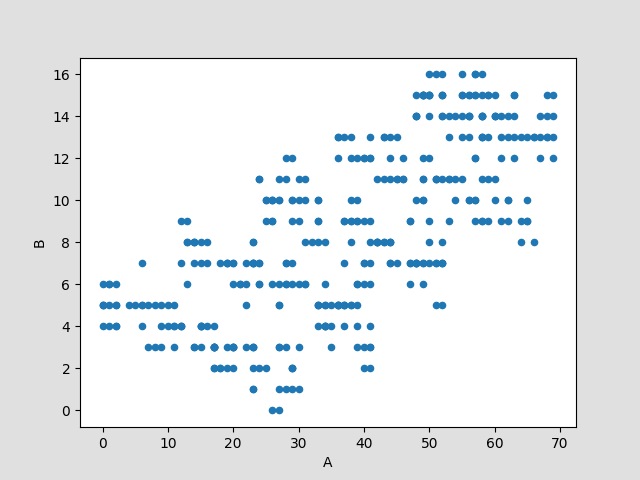the trend is increasing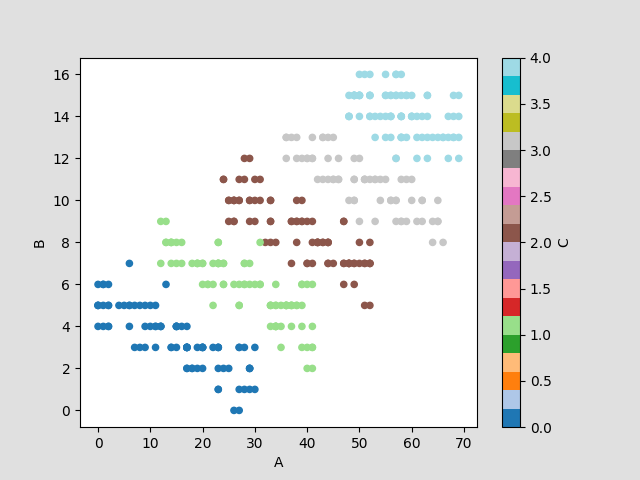the trend is decreasing for any value for C !

the Markov-equivalent model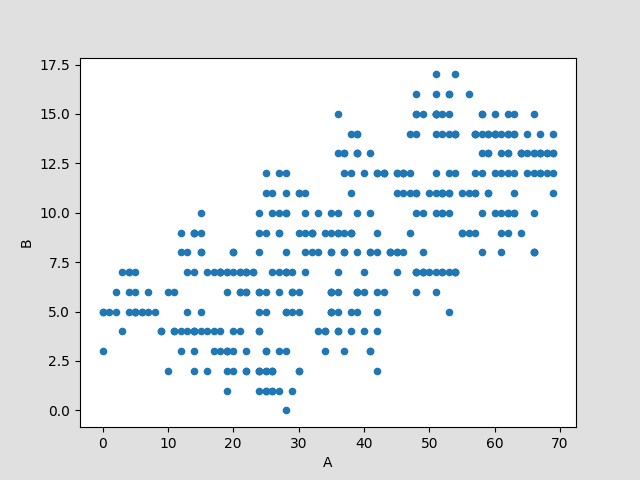the trend is increasing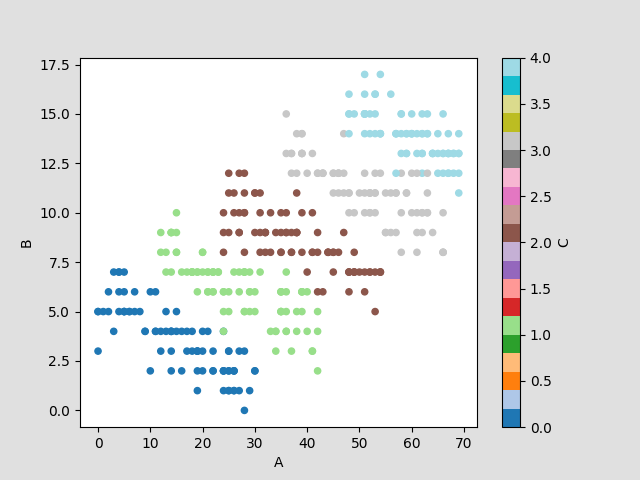the trend is decreasing for any value for C !

## The paradox is revealed in the trend of the inferred means : the means are increasing with the value of $$A$$except for any value of :math:C …

In :

for v in [10,20,30]:
gnb.flow.add_html(gnb.getPosterior(bn,target="B",evs={"A":v}),f"$P(B|A={v})$")
gnb.flow.new_line()
for v in [10,20,30]:
gnb.flow.add_html(gnb.getPosterior(bn,target="B",evs={"A":v,"C":0}),f"P(B | $A={v},C=0)$")
gnb.flow.new_line()
for v in [10,20,30]:
gnb.flow.add_html(gnb.getPosterior(bn,target="B",evs={"A":v,"C":2}),f"P(B | $A={v},C=2$)")
gnb.flow.new_line()
for v in [10,20,30]:
gnb.flow.add_html(gnb.getPosterior(bn,target="B",evs={"A":v,"C":4}),f"P(B | $A={v},C=4$)")
gnb.flow.display()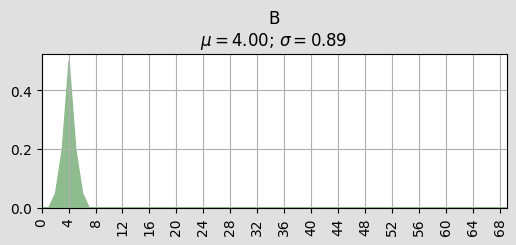$P(B|A=10)$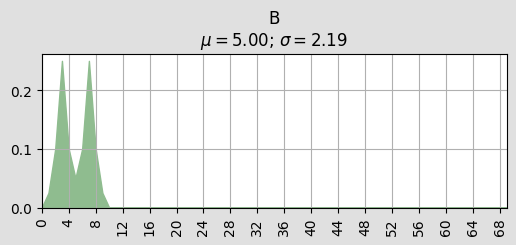$P(B|A=20)$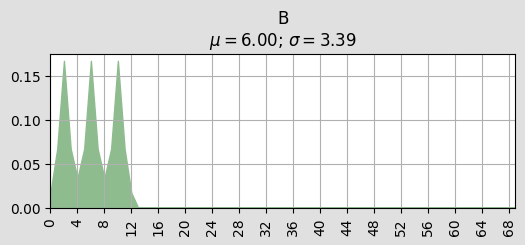$P(B|A=30)$P(B | $A=10,C=0)$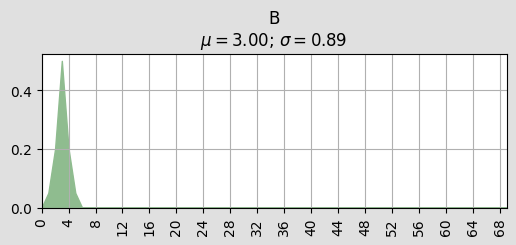P(B | $A=20,C=0)$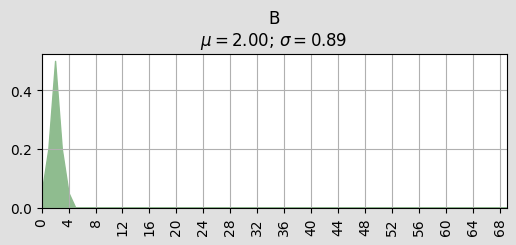P(B | $A=30,C=0)$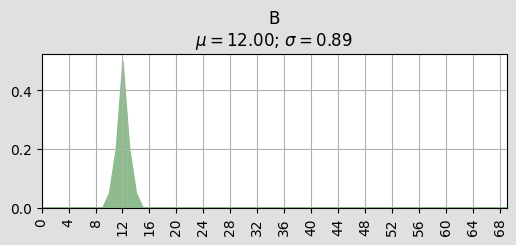P(B | $A=10,C=2$)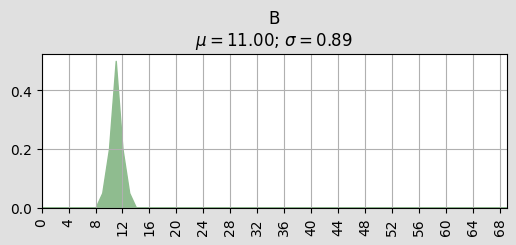P(B | $A=20,C=2$)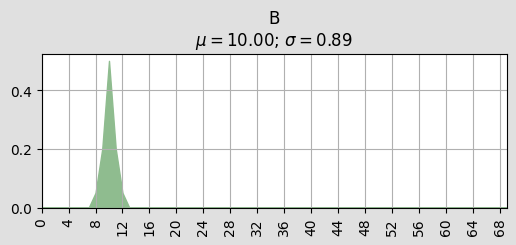P(B | $A=30,C=2$)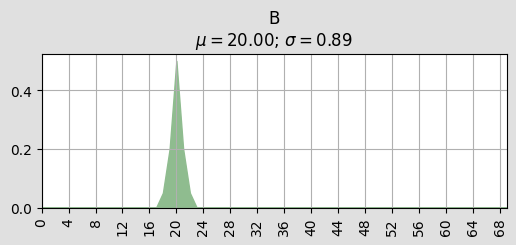P(B | $A=10,C=4$)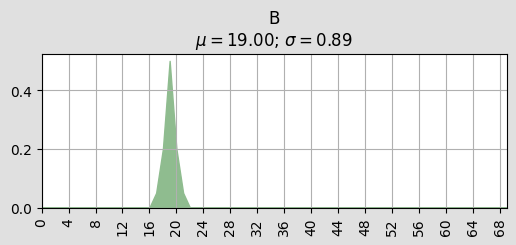P(B | $A=20,C=4$)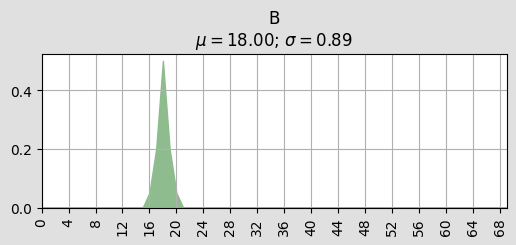P(B | $A=30,C=4$)

## Now that the paradoxal structure is understood and the paradox is revealed, will we choose to observe $$C$$ (or not) before deciding to increase or decrease $$A$$ (with the goal to maximize $$B$$) ?

Of course, it depends on the causal structure of the problem !

In :

gnb.flow.add_html(cslnb.getCausalModel(cm),"the first causal model")
for v in [10,20,30]:
gnb.flow.add_html(gnb.getProba(p.extract({'A':v})),f"Doing $A={v}$")
gnb.flow.display()


the first causal model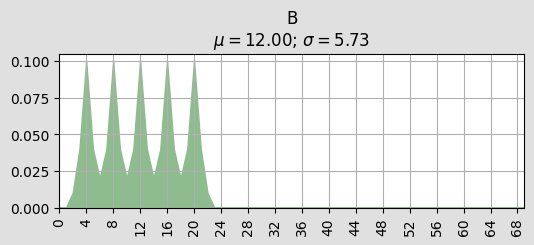Doing $A=10$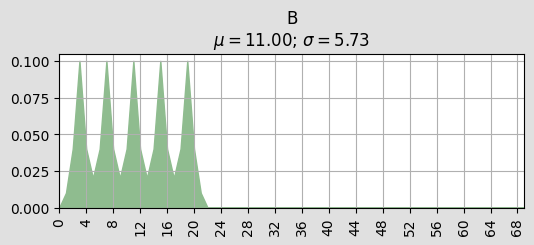Doing $A=20$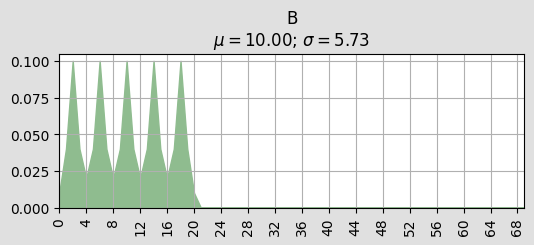Doing $A=30$

## If $$C$$ is cause for $$A$$, observing $$C$$ really gives a new information about $$B$$.

In :

gnb.flow.add_html(cslnb.getCausalModel(cm2),"the second causal model")
for v in [10,20,30]:
gnb.flow.add_html(gnb.getProba(p2.extract({'A':v})),f"Doing $A={v}$")
gnb.flow.display()


the second causal modelDoing $A=10$Doing $A=20$Doing $A=30$